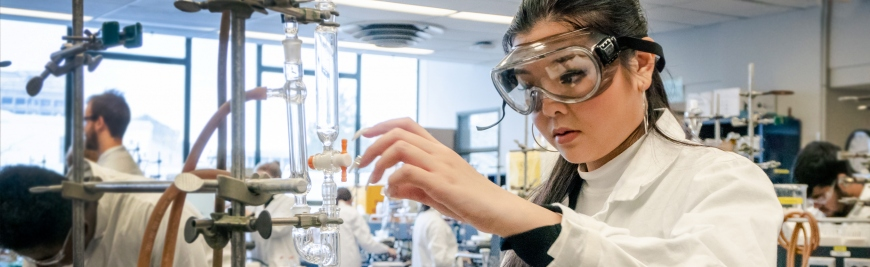## Research & Teaching Faculty# Experimental determination of the third derivative of G. I. Enthalpic interaction

 Title Experimental determination of the third derivative of G. I. Enthalpic interaction Publication Type Journal Article Year of Publication 2008 Authors Westh, P, Inaba, A, Koga, Y Journal Journal of Chemical Physics Volume 129 Pagination 4 Date Published Dec Type of Article Article ISBN Number 0021-9606 Keywords AQUEOUS-SOLUTIONS, calorimetry, DYNAMICS, enthalpy, fluctuations, H2O, HOFMEISTER SERIES, LIQUID MIXTURES, MOLECULAR-ORGANIZATION, organic compounds, SOLVATION, WATER Abstract The solute (i)-solute interaction in terms of enthalpy, H-i-i(E)=N(partial derivative H-2(E)/partial derivative n(i)(2))=(1-x(i))(partial derivative H-2(E)/partial derivative n(i)partial derivative x(i)), the third derivative of G, was experimentally determined using a Thermal Activity Monitor isothermal titration calorimeter for aqueous solutions of 2-butoxyethanol (BE) and 1-propanol (1P). This was done using both calorimetric reference and sample vessels actively. We simultaneously titrate small and exactly equal amounts of solute i (=BE or 1P) into both cells which contain the binary mixtures at an average mole fraction, x(i), which differs by a small amount Delta x(i). The appropriate amount of titrant delta n(i) was chosen so that the quotient (delta H-E/delta n(i)) can be approximated as (partial derivative H-E/partial derivative n(i)), and so that the scatter of the results is reasonable. delta H-E is the thermal response from an individual cell on titration, and we measure directly the difference in the thermal response between the two cells, Delta(delta H-E). The resulting quotient, Delta(delta H-E)/delta n(i)/Delta x(i), can be approximated as (partial derivative H-2(E)/partial derivative n(i)partial derivative x(i)) and hence provides a direct experimental avenue for the enthalpy interaction function. We varied the value of Delta x(i) to seek its appropriate size. Since H-E contains the first derivative of G with respect to T, the result is the third derivative quantity. Thus we present here a third derivative quantity directly determined experimentally for the first time. URL ://000261430900001